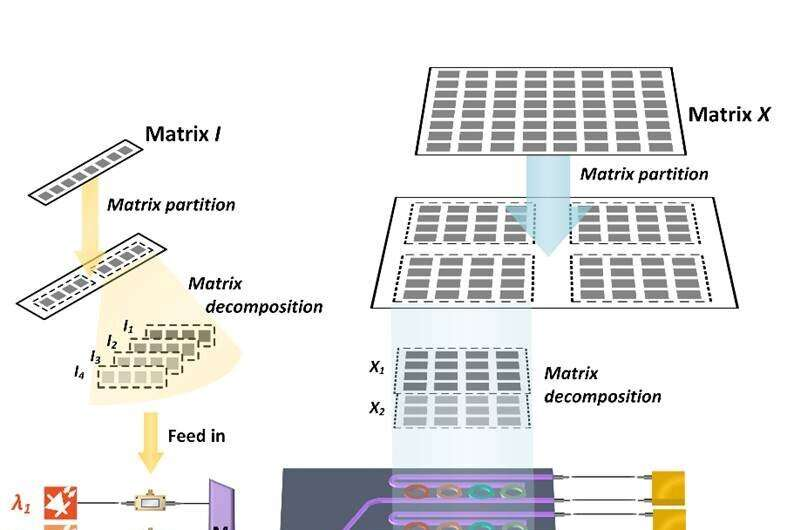# Small microring array enables large complex-valued matrix multiplication:Working principle of the photonic complex matrix-vector multiplier chip. Credit: Junwei Cheng, Yuhe Zhao, Wenkai Zhang, Hailong Zhou, Dongmei Huang, Qing Zhu, Yuhao Guo, Bo Xu, Jianji Dong, Xinliang Zhang ；

Optical computing uses photons instead of electrons to perform computations, which can significantly increase the speed and energy efficiency of computations by overcoming the inherent limitations of electrons. The basic principle of optical computing is the light-matter interaction. Matrix computing has become one of the most widely used and indispensable information processing tools in science and engineering, contributing a large number of computational tasks to most signal processing, such as discrete Fourier transforms and convolution operations.

As the basic building block of artificial neural networks (ANNs), matrix multiplication occupies most of the computational resources. Due to the properties of electronic components, performing simple matrix multiplications require a large number of transistors to work together, while matrix multiplications can be easily implemented by fundamental photonic components such as microrings, Mach Zehnder interferometers (MZIs), and diffractive planes. Therefore, the speed of optical computing is several orders of magnitude faster than electronic computing and consumes much less power. However, the traditional incoherent matrix-vector multiplication method focuses on real-valued operations and does not work well in complex-valued neural networks and discrete Fourier transforms.

Researchers led by Prof. Jianji Dong at Huazhong University of Science and Technology (HUST), China, have proposed a photonic complex matrix-vector multiplier chip that can support arbitrary large-scale and complex-valued matrix multiplications. The chip breaks the bottleneck of traditional non-coherent optical computing schemes, which have difficulty achieving arbitrary large-scale complex-valued matrix multiplications. It also enables artificial intelligence applications, such as discrete Fourier transforms, discrete cosine transforms, Walsh transforms and image convolutions.

Their idea is to design matrix decomposition and matrix partitioning intelligent algorithms for the microring array architecture to extend matrix multiplications from the real to the complex domain and from small scale to large scale. The researchers were successful at experimentally demonstrating several typical artificial intelligence applications, showing the potential of the photonic complex matrix-vector multiplier chip for applications in artificial intelligence computing. The work entitled “A small microring array that performs large complex-valued matrix-vector multiplication” was published on Apr. 28 2022 in: Frontiers of Optoelectronics:.

Speeding up machine learning by means of light: